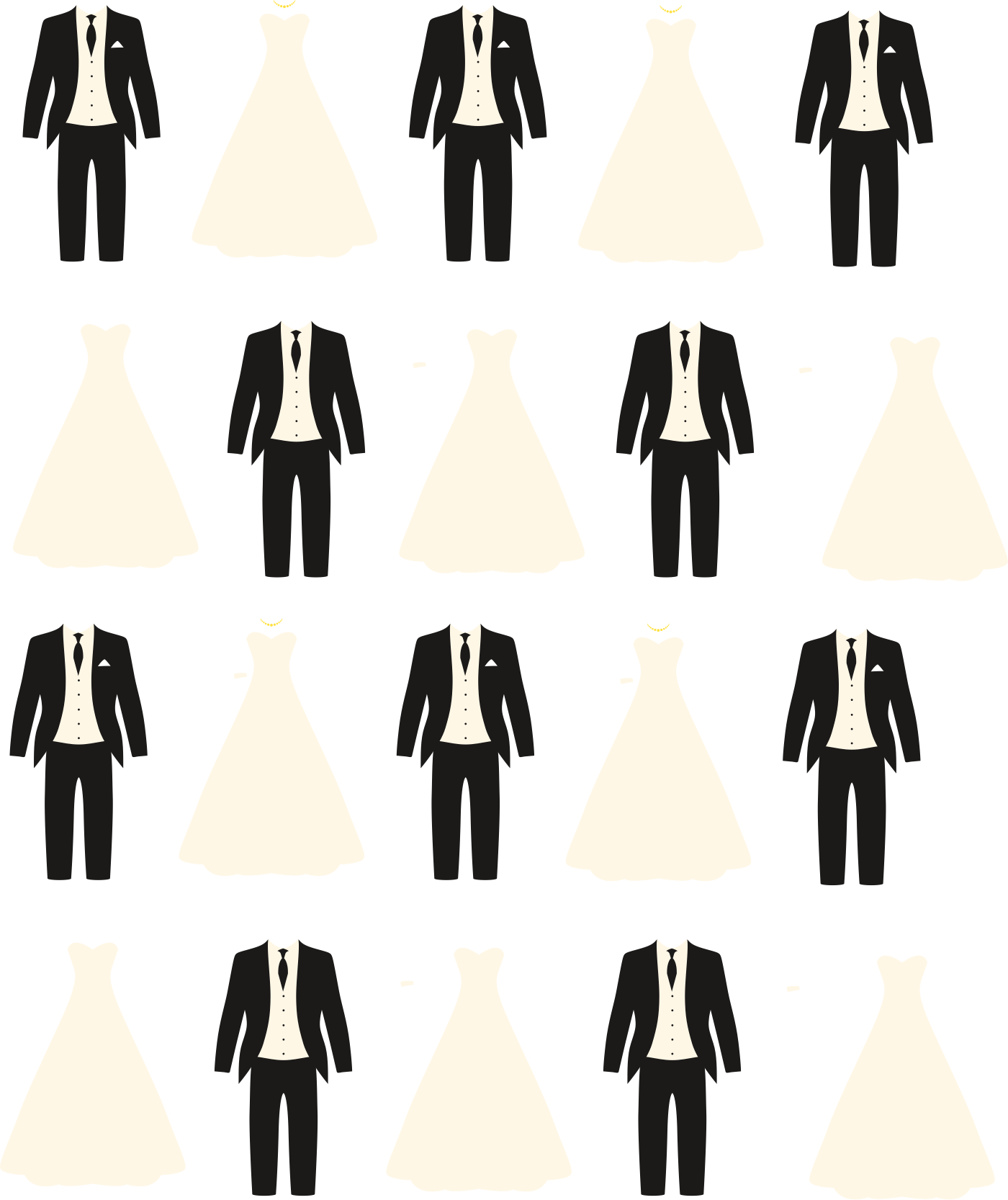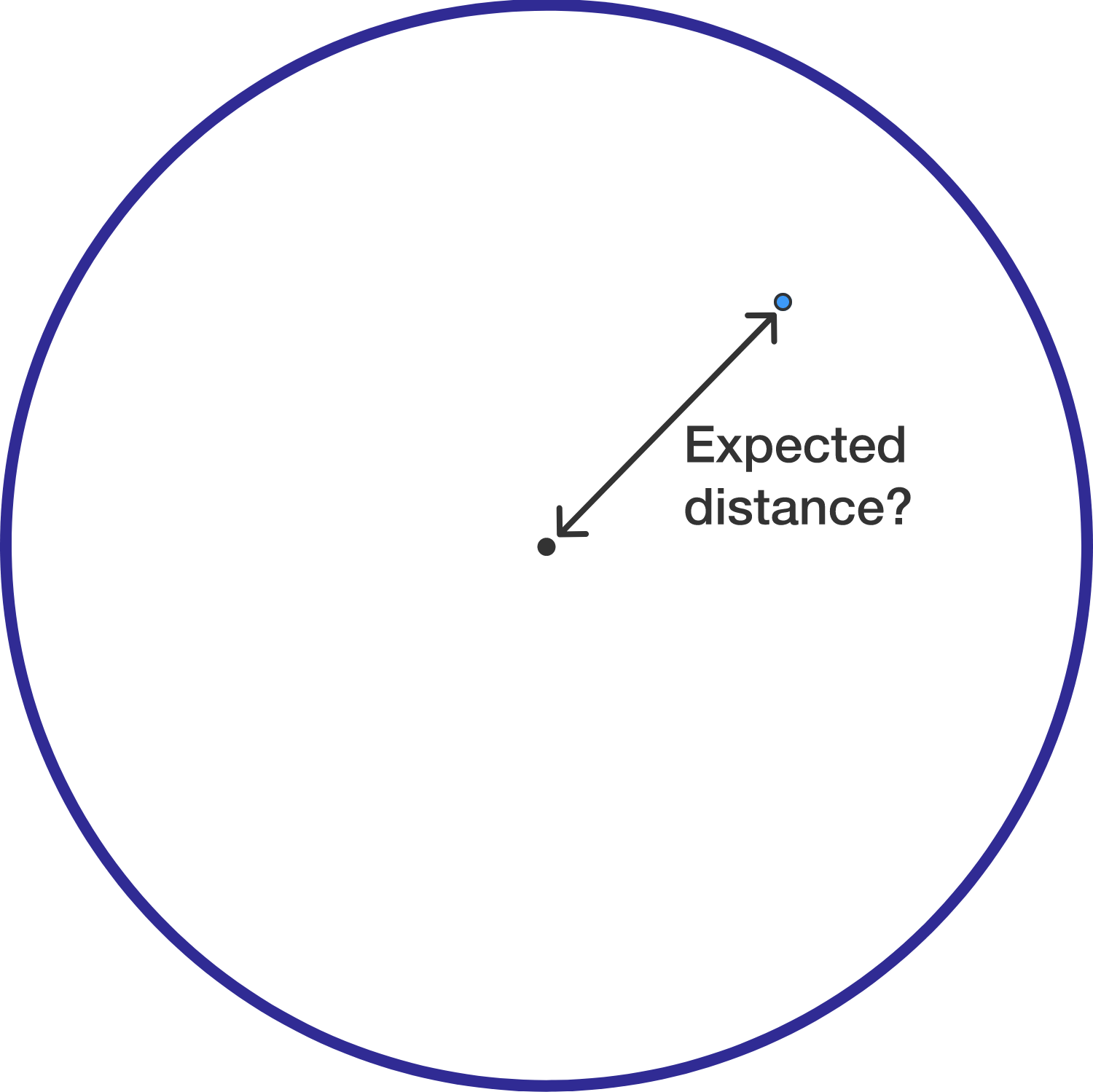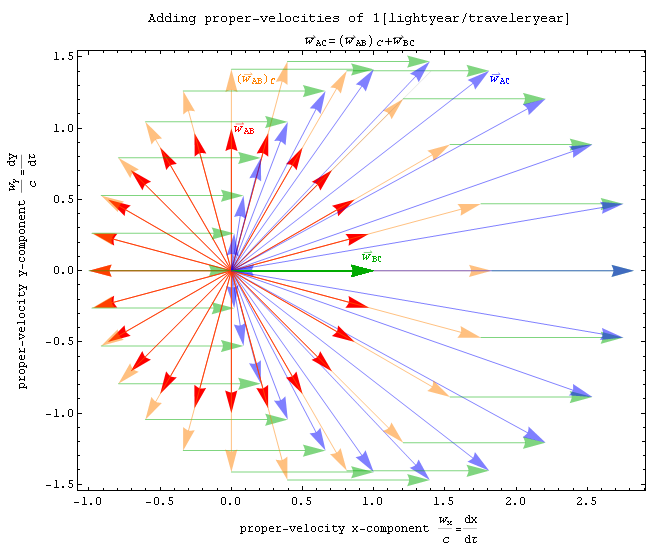Probability

# Expected Value: Level 4 ChallengesYou have 10 men and 10 women. Each man is married to one of the women (and no woman is married to more than one man). However, you don't know who is married to who; your job is to determine this.

To do this, you make several attempts. In an attempt, you match each man with some woman such that each woman is paired with exactly one man. Then, you are told which couples you guessed correctly. If you haven't guessed all couples correctly, you make another attempt. You repeat this until you get all correct.

Because you don't know anyone, your strategy is simple. You pick the couples at random. When you get a couple correct, in all future attempts, you will pair up that couple. For the other incorrect couples, you guess them at random again; this might mean you repeat a couple you previously attempted.

On average, how many attempts does it take for you to get all couples correct?A point is chosen randomly (by distribution of area) on the inside of a unit circle. Find the expected value of its distance from the center of the circle.

If you get your answer as $\dfrac{a}{b}$, where $a$ and $b$ are coprime positive integers, submit your answer as $a+b$.Two unit vectors in two-dimensional space $\hat{\textbf{a}}$ and $\hat{\textbf{b}}$ are added together. The expected magnitude of the resulting vector $\textbf{a+b}$ is equal to $E.$ What is $\lfloor100E\rfloor?$

Also try Daniel Liu's Expected Distance on a Circle.

###### Image credit: Wikipedia P.Fraundorf

For fixed integers $n$ and $k$, find all $k$-tuples of non-negative integers $(a_1, a_2, \ldots a_k)$ such that

$a_1 + a_2 + \cdots + a_k = n$

For each integer $i$ from 0 to $n$, let $p_i$ be the probability that one of the $a_j$ is equal to $i$. What is the value of

$1\times p_1 + 2 \times p_2 + \cdots n \times p_n ?$

I am feeling sleepy but can't sleep. So I decide to roll a dice as many times as it takes until I get a 6 immediately after a 5.

Assuming each throw takes me one second and I will fall asleep as soon as I get a 6 immediately after a 5, what is the expected time (in seconds) in which I will fall asleep?

For example, if the results of the dice rolls are ${1, 6, 5, 6, 5, 2}$, then I must stop at roll 4 and fall asleep immediately, which implies that the time taken would be 4 seconds.

×